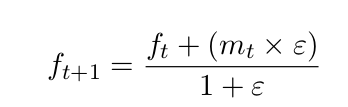# Department of Computer Science

https://www.univ-soukahras.dz/en/dept/cs

Module: Initiation à la recherche

1. Information
2. Questions
3. E-Learning

## Writing Equations using Latex (4)

 4 votes What's the latex code for the following Equation:Asked on 19:29, Monday 16 May 2016 By Imed BOUCHRIKA In Initiation à la recherche

 2 votes \begin{equation}  f_{t+1} = \frac{f_{t}+(m_{t}\times \epsilon)}{1+\epsilon}\end{equation} Answered on 20:53, Monday 16 May 2016 by mohammed tebib(377 points) In Initiation à la recherche

 1 votes \begin{frame}     \begin{equation}         f_{t+1} = \frac{f_{t} + (m_{t) \times \epsilon}}{1+\epsilon}      \end{equation} \end{frame} mais l'epsilon de numérateur est un indice Answered on 21:36, Monday 16 May 2016 by Hanen Rouainia(322 points) In Initiation à la recherche
 0 votes \begin{equation}f_{t+1} = \frac {f_t + (m_t \times \epsilon )} { 1 + \epsilon}\end{equation} Answered on 20:03, Friday 27 May 2016 by amirat abdallah(257 points) In Initiation à la recherche